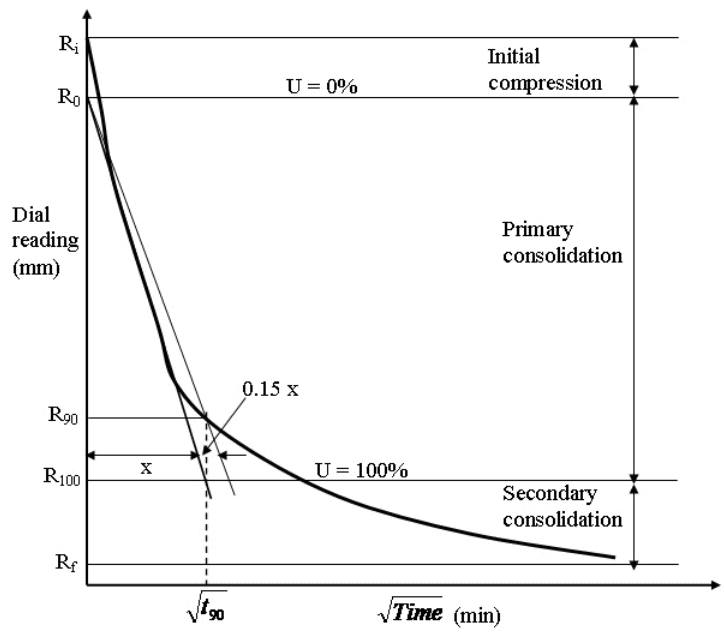Taylor’s Square Root of Time Fitting Method - Determination of Coefficient of Consolidation of Soil,

# Taylor’s Square Root of Time Fitting Method - Determination of Coefficient of Consolidation of Soil, - Notes | Study Soil Mechanics Notes- Agricultural Engineering - Agricultural Engineering

 1 Crore+ students have signed up on EduRev. Have you?

Taylor’s Square Root of Time Fitting Method

From the oedometer test (explained in Lesson 23) the dial reading (settlement) corresponding to a particular time is measured.  From the measured data, dial reading vs $\sqrt {Time}$ graph can be drawn (as shown in Figure 24.1). A straight line can be drawn passing through the points on initial straight portion of the curve (as shown in Figure 24.1). The intersection point between the straight line and the dial reading axis is denoted as R0 which is corrected zero reading i.e U = 0%.  Starting from R0, draw another straight line such that its abscissa is 1.15 times the abscissa of first straight line. The intersection point between the second straight line and experimental curve represents theR90 and corresponding $\sqrt {{t_{90}}}$ is determined. Thus, the time required (t90) for 90% consolidation is calculated. The Coefficient of consolidation (cv) is determined as:

${c_v}={{{T_v}{H^2}} \over t}$                    (24.1)

where H is the thickness of the soil sample, t is required time. Tv is the vertical time factor and can be determine as:

${T_v}=\left({{\pi\over 4}}\right){U^2}\quad \quad if\;U \le 60\%$   %                (24.2)

${T_v}=1.781 - 0.933{\log _{10}}(100 - U)\quad \quad if\;U > 60\%$   %               (24.3)Fig. 24.1. Taylor’s Square Root of Time Fitting Method.

The document Taylor’s Square Root of Time Fitting Method - Determination of Coefficient of Consolidation of Soil, - Notes | Study Soil Mechanics Notes- Agricultural Engineering - Agricultural Engineering is a part of the Agricultural Engineering Course Soil Mechanics Notes- Agricultural Engineering.
All you need of Agricultural Engineering at this link: Agricultural Engineering

## Soil Mechanics Notes- Agricultural Engineering

64 docs
 Use Code STAYHOME200 and get INR 200 additional OFF

## Soil Mechanics Notes- Agricultural Engineering

64 docs

Track your progress, build streaks, highlight & save important lessons and more!

,

,

,

,

,

,

,

,

,

,

,

,

,

,

,

,

,

,

,

,

,

,

,

,

;